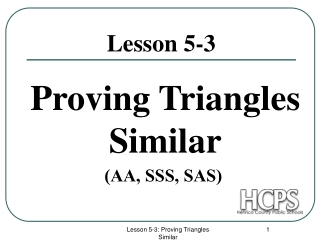DownloadDownload PresentationLesson 5-3

# Lesson 5-3

Download Presentation## Lesson 5-3

- - - - - - - - - - - - - - - - - - - - - - - - - - - E N D - - - - - - - - - - - - - - - - - - - - - - - - - - -
##### Presentation Transcript

1. Lesson 5-3 Proving Triangles Similar (AA, SSS, SAS) Lesson 5-3: Proving Triangles Similar

2. AA Similarity (Angle-Angle) If 2 angles of one triangle are congruent to 2 angles of another triangle, then the triangles are similar. Given: and Conclusion: Lesson 5-3: Proving Triangles Similar

3. 5 10 16 8 11 22 SSS Similarity (Side-Side-Side) If the measures of the corresponding sides of 2 triangles are proportional, then the triangles are similar. Given: Conclusion: Lesson 5-3: Proving Triangles Similar

4. 5 10 11 22 SAS Similarity (Side-Angle-Side) If the measures of 2 sides of a triangle are proportional to the measures of 2 corresponding sides of another triangle and the angles between them are congruent, then the triangles are similar. Given: Conclusion: Lesson 5-3: Proving Triangles Similar

5. Similarity is reflexive, symmetric, and transitive. Proving Triangles Similar Steps for proving triangles similar: 1. Mark the Given. 2. Mark … Shared Angles or Vertical Angles 3. Choose a Method. (AA,SSS , SAS) Think about what you need for the chosen method and be sure to include those parts in the proof. Lesson 5-3: Proving Triangles Similar

6. G D C E F Problem #1 Step 1: Mark the given … and what it implies Step 2: Mark the vertical angles AA Step 3: Choose a method: (AA,SSS,SAS) Step 4: List the Parts in the order of the method with reasons Step 5: Is there more? Given Alternate Interior <s Alternate Interior <s AA Similarity Lesson 5-3: Proving Triangles Similar

7. Problem #2 Step 1: Mark the given … and what it implies SSS Step 2: Choose a method: (AA,SSS,SAS) Step 4: List the Parts in the order of the method with reasons Step 5: Is there more? 1.IJ = 3LN ; JK = 3NP ; IK = 3LP Given Division Property Substitution SSS Similarity Lesson 5-3: Proving Triangles Similar

8. Problem #3 Step 1: Mark the given … and what it implies Step 2: Mark the reflexive angles SAS Step 3: Choose a method: (AA,SSS,SAS) Step 4: List the Parts in the order of the method with reasons Next Slide…………. Step 5: Is there more? Lesson 5-3: Proving Triangles Similar

9. Lesson 5-3: Proving Triangles Similar